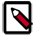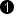## Operator MULT

Operator MULT multiplies the values at input link I1 by the values at input link I2. At output link O, the result of the multiplication is provided.

Operator MULT supports asymmetric arithmetic types on its inputs, i.e., SIGNED and UNSIGNED may be mixed up so that you can multiply an unsigned multiplier with a signed multiplicand or a signed multiplier with an unsigned multiplicand as well as a signed multiplier with a signed multiplicand or an unsigned multiplier with an unsigned multiplicand.

ResourcesA multiplication requires many FPGA resources. For scaling with a constant use operator SCALE or ShiftLeft instead. Moreover, most frame grabbers include embedded arithmetic logic units (embedded multipliers). Use operator HWMULT to save resources.

### I/O Properties

Property Value
Operator Type O
I2, multiplicand 2

Bit Width [1, 32] unsigned, [2, 32] signed [1, 32] unsigned, [2, 32] signed autoArithmetic {unsigned, signed} as I as I
Parallelism any as I as I
Kernel Columns any as I as I
Kernel Rows any as I as I
Img Protocol {VALT_IMAGE2D, VALT_LINE1D, VALT_PIXEL0D} as I as I
Color Format VAF_GRAY as I as I
Color Flavor FL_NONE as I as I
Max. Img Width any as I as I
Max. Img Height any as I as I
 The output bit width is the sum of the input bit widths i.e. bit width at I1 + bit width at I2.

None

### Examples of Use

The use of operator MULT is shown in the following examples: